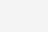# Python使用技巧：一行代码可减少一半的内存占用！## 图片的结果class DataItem(object):
self.name = name
self.age = age

## 初学者的问题：如何知道一个以上这样的对象占用多少内存？

d1 = DataItem(“Alex”42“-“)
print (“sys.getsizeof(d1):”, sys.getsizeof(d1))

d2 = DataItem(“Boris”24“In the middle of nowhere”)
print (“sys.getsizeof(d2):”, sys.getsizeof(d2))

• 直觉不会让我们失望，一切都不是那么简单。Python是一种具有动态类型的非常灵活的语言，对于它的工作，它存储了大量的附加数据。它们本身占据了很多。

## 这个类，占多少内存？

def dump(obj):
for attr in dir(obj):
print(”  obj.%s = %r” % (attr, getattr(obj, attr)))## 这一切内容占用多少内存？

def get_size(obj, seen=None):
# From
# Recursively finds size of objects
size = sys.getsizeof(obj)
if seen is None:
seen = set()
obj_id = id(obj)
if obj_id in seen:
return 0
# Important mark as seen *before* entering recursion to gracefully handle
# self-referential objects
if isinstance(obj, dict):
size += sum([get_size(v, seen) for v in obj.values()])
size += sum([get_size(k, seen) for k in obj.keys()])
elif hasattr(obj, ‘__dict__’):
size += get_size(obj.__dict__, seen)
elif hasattr(obj, ‘__iter__’and not isinstance(obj, (str, bytes, bytearray)):
size += sum([get_size(i, seen) for i in obj])
return size

d1 = DataItem(“Alex”42“-“)
print (“get_size(d1):”, get_size(d1))
d2 = DataItem(“Boris”24“In the middle of nowhere”)
print (“get_size(d2):”, get_size(d2))

## 是否存在减少内存开销的可能呢？

d1 = DataItem(“Alex”42“-“)
print (“get_size(d1):”, get_size(d1))
d1.weight = 66
print (“get_size(d1):”, get_size(d1))

class DataItem(object):
self.name = name
self.age = age

data = []
for p in range(100000):
data.append(DataItem(“Alex”, 42, “middle of nowhere”))
snapshot = tracemalloc.take_snapshot()
top_stats = snapshot.statistics(‘lineno’)
total = sum(stat.size for stat in top_stats)
print(“Total allocated size: %.1f MB” % (total / (1024*1024)))

def toJSON(self):
return json.dumps(self.__dict__)

def toJSON(self):
data = dict()
for var in self.__slots__:
data[var] = getattr(self, var)
return json.dumps(data)

## 没有 __slots__:6.9Mb 变成 27Mb … 好家伙, 毕竟, 我们节省了内存, 27Mb 代替 70 ，对于增加一行代码来说并不是一个坏的例子